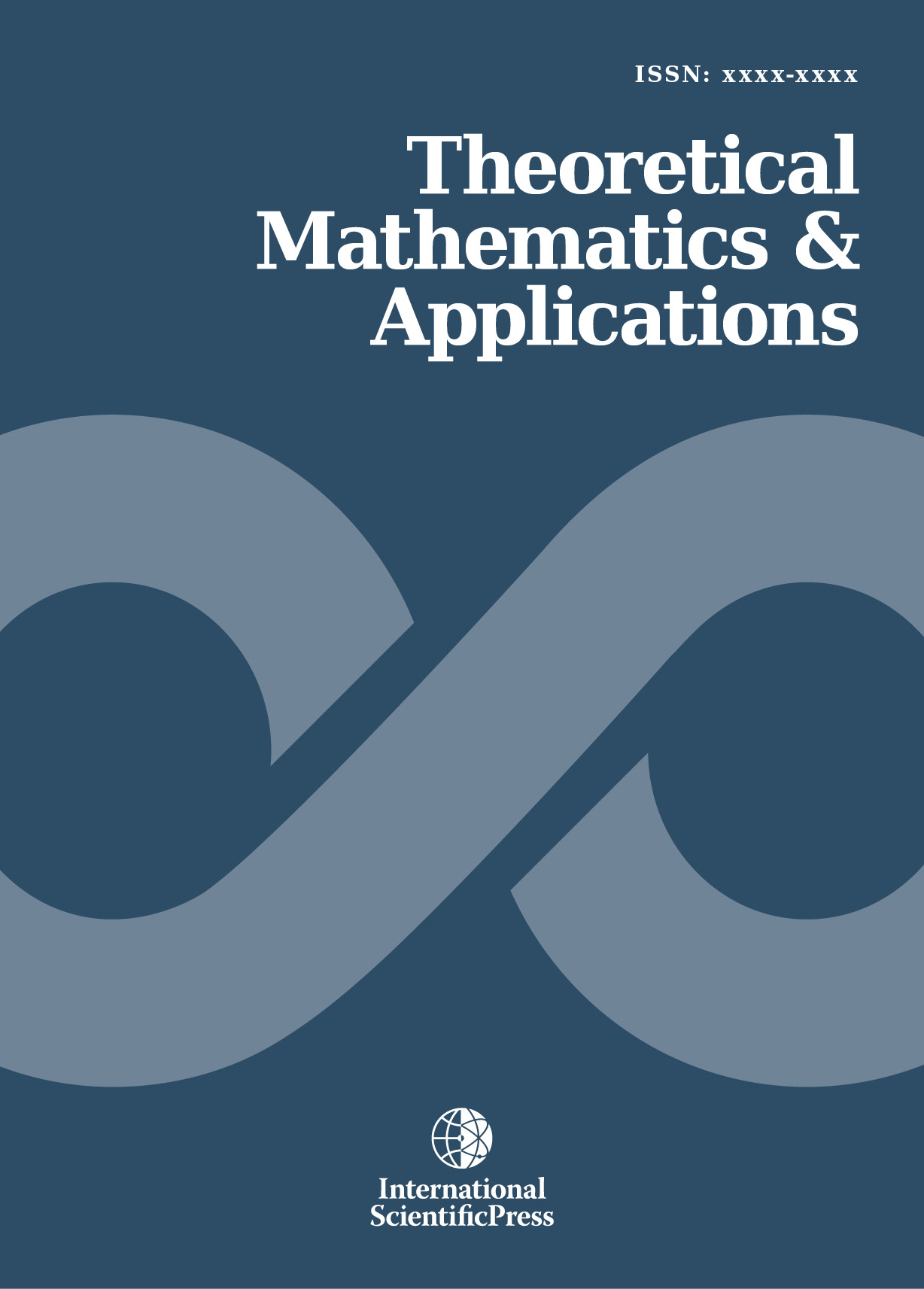# Theoretical Mathematics & Applications

#### Extended Adomian’s polynomials for solving non-linear fractional differential equations

•[ Download ]
• Times downloaded: 1067
•                                                  Abstract

In this paper, we implement the improved Adomian’s polynomials introduced by Abassy in 2010 with an extension to solve numerically the non-linear initial value problems of fractional differential equations (FDEs). This proposed extension is called extended Adomian’s polynomials. An adaption of the convergence analysis which was introduced by Hosseini and Nasabzadeh in 2006 is formulated to be used to prove that these polynomials accelerate the convergence rate of the series solution comparing with the standard Adomian’s polynomials. Also, we use the so called improved Adomian decomposition method (IADM) as a special case of the proposed method where the fractional derivative  α = 1. A comparison is made between IADM and ADM for some examples to illustrate the efficiency of the proposed treatment for non-linear initial value problems of FDEs.ISSN: 1792-9687 (Print)
1792-9709 (Online)